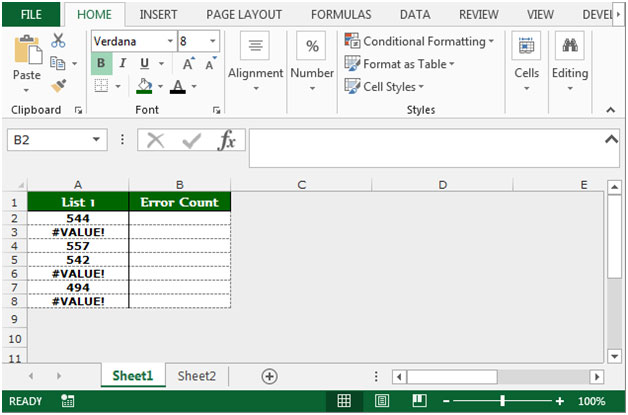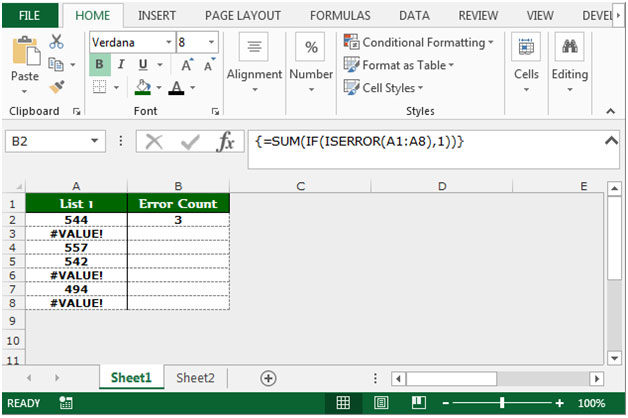# Count the number of cells in a range containing all types of Errors in Microsoft Excel

In this article, we will learn counting the number of cells in a range containing all types of errors in Microsoft Excel. We will use ISERROR  function along with  SUM and IF functions.

ISERROR  function is used to check whether a value is an error (#N/A, #VALUE!, #REF!, #DIV/0!, #NUM!, #NAME?, OR #NULL!) and returns True or False.

SUM function is used for adding all the numbers in a range or cells.

IF function checks whether a condition is met and returns one value if True and another value if False.

Let’s take an example to understand:-

We have a list of numbers in range A1:A8. In the list, some cells contain the Error. To count the errors in a list, follow the below given steps:-• Write the formula in cell B2.
• =SUM(IF(ISERROR(A1:A8),1))
• Press Ctrl+Shift+Enter on your keyboard.
• After pressing the key, the formula will look like {=SUM(IF(ISERROR(A1:A8),1))}.
• The function will return the total count of errors.Note: By using this formula, we can count the number of errors. We can also get a count of different types of errors. This is the way by which we count errors by using the ISERROR formula along with SUM and IF functions in Microsoft Excel.We would love to hear from you, do let us know how we can improve, complement or innovate our work and make it better for you. Write us at info@exceltip.com

1.You can avoid using an array formula by using SUMPRODUCT.

=SUMPRODUCT(--ISERROR(\$A\$1:\$B\$15))

•That works wonders... although I have no Idea why it works!

Terms and Conditions of use

The applications/code on this site are distributed as is and without warranties or liability. In no event shall the owner of the copyrights, or the authors of the applications/code be liable for any loss of profit, any problems or any damage resulting from the use or evaluation of the applications/code.